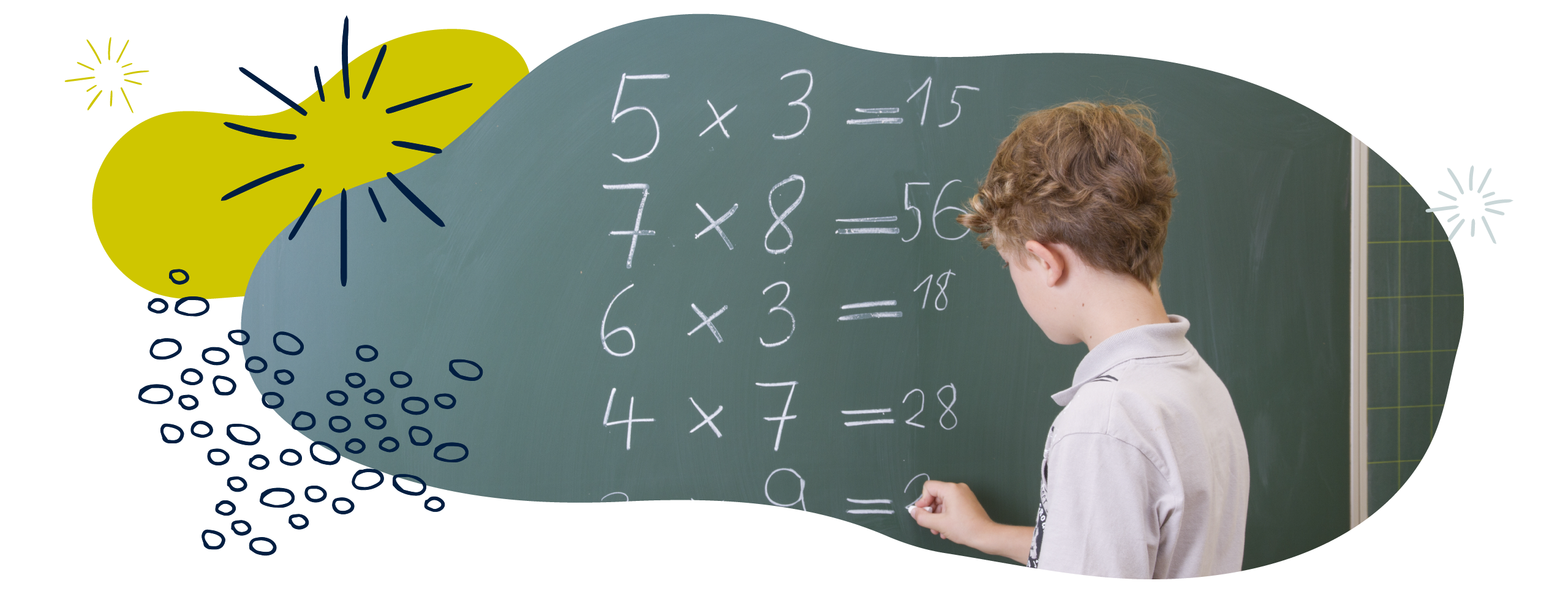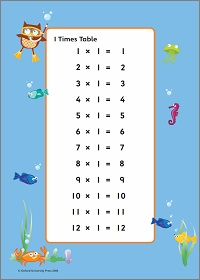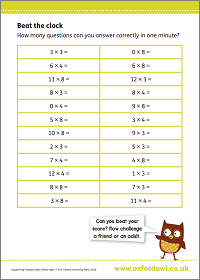We use cookies to enhance your experience on our website. By continuing to use our website, you are agreeing to our use of cookies. You can change your cookie settings at any time. Find out more# Multiplication & division in Year 3 (age 7–8)

In Year 3, your child will learn to multiply a two-digit number by a one-digit number, such as 45 × 3, using a range of mental and informal methods. They will use objects, drawings, diagrams, and formal written methods.

The key words for this section are array and inverse operations.

## What your child will learn

Take a look at the National Curriculum expectations for multiplication and division in Year 3 (ages 7–8):

#### Learn the 3, 4, and 8 times tables

Your child will be expected to know multiplication facts for the 3, 4, and 8 times tables. They will use this knowledge to work out division facts – for example, if 3 × 4 = 12 then 12 ÷ 4 = 3 and 12 ÷ 3 – 4. Your child will also use doubling to connect the 2, 4, and 8 times tables and use their knowledge of times tables to work out other multiplication and division facts.

Your child will be able to tell the difference between odd and even numbers.

#### Solve calculations using mental and written methods

In Year 3, your child will learn to multiply a two-digit number by a one-digit number, such as 45 × 3, using a range of mental and informal methods. These might include using objects, drawings, or diagrams (such as the area/grid method for multiplication and chunking for division).

They will use formal written methods such as column multiplication and column division.

#### Solve scaling problems and correspondence problems

Your child will continue solving multiplication problems using their knowledge of times tables.

They will understand multiplication as scaling. For example:

My chocolate bar is 4cm long. Your chocolate bar is three times as long as mine. How long is your chocolate bar?

They will also be expected to solve correspondence problems. For example:

If I had 3 hats and 4 coats, how many different outfits could I wear?

Your child will be expected to use their knowledge of inverse operations to solve missing number problems. For example, to solve 72 ÷ ? = 4, children could use their knowledge that 8 × 9 = 72, so 4 × 18 = 72, so the missing value is 18.

#### Solve problems in contexts using lots of different methods

Your child will learn a variety of methods to calculate multiplication and division problems. For multiplication, these might include:

• Counting in steps of 3, 4, or 8 mentally
• Using objects or drawing pictures
• Using number lines to count forwards in steps
• Using the bar model or area/grid method.

Children may solve division problems by:

• Sharing or grouping objects equally
• Using a number line to count backwards in steps of 3, 4, or 8
• Using their understanding of inverse to use multiplication to work out division problems. For example, when solving 8 ÷ 2, children may count in steps of two to find out how many groups of 2 there are in 8.

Your child will be expected to choose a method to solve a problem and to be able to check their answer using a different method. This is why it is important that children learn a range of methods for solving problems.

There is also an expectation that they will be able to explain how they have solved a problem and why they have chosen a particular method.

## How to help at home

There are lots of quick and easy ways that you can help your child to understand multiplication and division. Here are just a few ideas to support your child’s learning:

### 1. Practise times tables

Encourage your child to practise multiplication facts from the 2, 3, 4, 5, 8, and 10 times tables. Why not make or print out multiplication facts for the times tables your child is learning? Lay the cards out face down in front of your child. Ask them to choose a card and work out the problem. They can win a point for each one they get right.

If your child is struggling to work out the answer, you could support them by asking them if they know any other facts that may help them to work it out. For example, if they are struggling to work out 5 × 9, ask them if they know the solution to 5 × 10, and see if they can use the answer to help them.

You could also help your child by showing them real-life examples of a times table. For example, a muffin tin will normally have four rows of three muffin cups each, showing the multiplication 4 × 3. Find opportunities to sing and chant times tables together, for example, in the car or on the walk to school.

### Activity: Times tables wall chartsCharts for all times tables from 1–12.

### Activity: Beat the clockAnswer as many as you can in one minute.

### 2. Think about multiplication as scaling

You can also help your child by introducing the idea of multiplication as scaling in the real world. Scaling is used when we use multiplication to change the size of the original quantity. For example:

‘If you have saved £32 and I have saved three times as much as you, how much money have I saved?’

‘If you are 5 years old and I am 6 times older than you, how old am I?’

### 3. Use different methods

Your child will be taught a range of mental and informal methods to solve multiplication problems at school, as well as more formal methods including column multiplication.

To practise these methods, choose a multiplication problem and have you and your child work out the answer out independently. Compare your methods. Did you use the same strategies to solve the problem? Try each other’s method. Discuss which one you both preferred and why.

Are there any other ways we could have worked out the answer? Being able to use several methods will mean they can always use the best one for any given question. By practising lots of different ways of multiplying numbers, you help them gain confidence with formal and informal methods.

### 4. Use arrays

Arrays are shapes or objects arranged in a rectangle. Muffin trays and egg boxes are examples of arrays.

Give your child a specific number of objects and ask them to make an array. When they have made an array, ask your child to write as many calculations as they can using the array. For example, using 12 counters, your child could make the following array:The following calculations could be made using this array:

4 + 4 + 4 = 12

3 + 3 + 3 + 3 = 12

4 × 3 = 12

3 × 4 = 12

12 ÷ 4 = 3

12 ÷ 3 = 4

Challenge your child to make as many arrays as they can using the 12 counters.

### 5. Find connections in multiplication and division

Being able to make connections between multiplication and division is helpful for solving problems. Encourage your child to explore the connections between multiplication, arrays, and repeated addition. In terms of division, encourage your child to:

• Explore grouping and sharing structures of division and arrays
• Explore the relationship between division and repeated subtraction
• See the connections between fractions and division (for example, 40 ÷ 2 = 20, so 20 is half of 40).